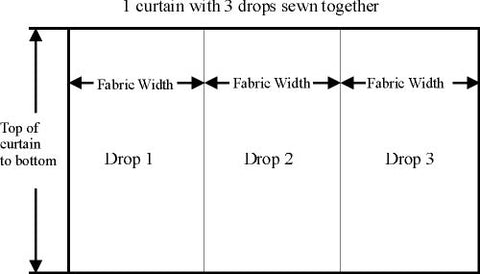# Calculating fabric for curtains

To calculate the amount of fabric needed for curtains by drops:For drops the fabric width is less than the width of the window and needs to be joined together. The following formula is used for the Easy Track system to calculate how much fabric is needed when fabric is used by the drop: 1. Calculate number of drops: Lenght of track x Fullness(2:1) + Allow- ance(40cm) / Fabric Width then round up answer to nearest drop. Example 200cm x 2 + 40cm = 440cm / 140 = 3.14 roundup to 4 drops. 2. Calculate lenght of each drop: Distance under track to foor - allowance for hanging glider hanging below track and amount above floor (1.5cm + 1.5cm = 3cm) + Allowance for hems(30cm). Example 213cm - 3cm + 30cm = 240cm fabric required per drop. 2A. If there is a pattern repeat(PR): Devide the fabric per drop calculated without pattern repeat in step to by the by pattern repeat, round the devided number up to the nearest whole number and multiply number by pattern repeat. Example fabric per drop with no pattern repeat = 240cm / 37cm(PR) = 6.486 round up to 7 = 7 x 37cm(PR) = 259cm fabric required per drop with PR 37cm 3. Multiply number of drops calculated for window in step 1 x fabric required in step 2. Example 4 drops x 240cm = 960cm or 9.6 metres

To calculate the amount of fabric required for continuous curtain - normally sheers or lining curtain:For continuous the fabic that is wider than the curtain is going to be and is made in one piece: 1. Calculate number width needed: Lenght of track x Fullness(2.5:1) + Allow- ance(40cm). Example 200cm x 2.5 + 40cm = 540cm or 5.4 metres. 2. Because the fabric is continuous no more calculations need to be done the amount needed is simply the track width x the fullness + Allowance. To calculate the amount of continuous lining required to be attached behind a curtain: 1. Number of drops in curtain x fabric width = lining required. Example 2 drops x 140cm(Fabric width) = 280cm continuous lining reqired.